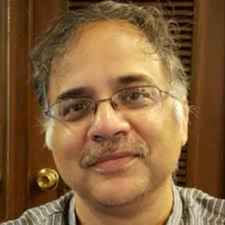X
Solving dual problems using a coevolutionary optimization algorithm
K. Deb, S. Gupta, B. Ranjan
Published in
2013
Volume: 57

Issue: 3
Pages: 891 - 933
Abstract
In solving certain optimization problems, the corresponding Lagrangian dual problem is often solved simply because in these problems the dual problem is easier to solve than the original primal problem. Another reason for their solution is the implication of the weak duality theorem which suggests that under certain conditions the optimal dual function value is smaller than or equal to the optimal primal objective value. The dual problem is a special case of a bilevel programming problem involving Lagrange multipliers as upper-level variables and decision variables as lower-level variables. Another interesting aspect of dual problems is that both lower and upper-level optimization problems involve only box constraints and no other equality of inequality constraints. In this paper, we propose a coevolutionary dual optimization (CEDO) algorithm for co-evolving two populations - one involving Lagrange multipliers and other involving decision variables - to find the dual solution. On 11 test problems taken from the optimization literature, we demonstrate the efficacy of CEDO algorithm by comparing it with a couple of nested smooth and nonsmooth algorithms and a couple of previously suggested coevolutionary algorithms. The performance of CEDO algorithm is also compared with two classical methods involving nonsmooth (bundle) optimization methods. As a by-product, we analyze the test problems to find their associated duality gap and classify them into three categories having zero, finite or infinite duality gaps. The development of a coevolutionary approach, revealing the presence or absence of duality gap in a number of commonly-used test problems, and efficacy of the proposed coevolutionary algorithm compared to usual nested smooth and nonsmooth algorithms and other existing coevolutionary approaches remain as the hallmark of the current study. © 2012 Springer Science+Business Media New York.
•